# 学会使用PHP的List、each函数及配合list函数与each函数是我们在日常生活中经常能够用到的函数，那么这两个函数应该怎么使用？两者之间有什么特点？他们又是怎么配合使用的？这两个函数是比较有意思的数组函数，函数学习起来并不难，那我们一起来看看吧。

list函数

`list`函数它的功能是：能够将索引数组下标为0的对应为变量1，下标为1的对应变量2…list函数能够将数组的元素变成变量使用。

`list(变量1,变量2...)`

```<?php
list(\$a , \$b , \$c, \$d) = array('好好学习' ,'天天向上' ,'福如东海','寿比南山');
echo '\$a----'.\$a.'<br />';
echo '\$b----'.\$b.'<br />';
echo '\$c----'.\$c.'<br />';
echo '\$d----'.\$d.'<br />';
?>```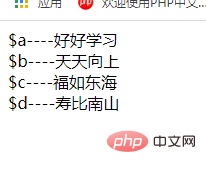```<?php
list( ,  , \$c, \$d) = array('好好学习' ,'天天向上' ,'福如东海','寿比南山');
echo '\$a----'.\$a.'<br />';
echo '\$b----'.\$b.'<br />';
echo '\$c----'.\$c.'<br />';
echo '\$d----'.\$d.'<br />';
?>```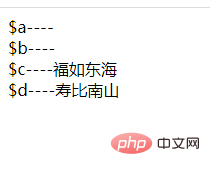```<?php
list(\$a , \$b , \$c, \$d) = array(2=>'好好学习' ,'天天向上' ,'福如东海','寿比南山');
echo '\$a----'.\$a.'<br />';
echo '\$b----'.\$b.'<br />';
echo '\$c----'.\$c.'<br />';
echo '\$d----'.\$d.'<br />';
?>```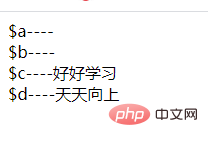each函数

`each`函数的功能是传入一个数组。它会将其中的一个元素拆分为一个新的数组。每次执行这样操作一个元素。执行一次先后移动一次，同样的方式操作下一个数组元素。执行到最后，返回false。

```<?php
\$a=[
'aaa'=>'好好学习',
'bbb'=>'天天向上',
'ccc'=>'福如东海',
'ddd'=>'寿比南山'
];
//第一次each
\$data = each(\$a);
echo '<pre>';
var_dump(\$data);
echo '</pre>';
?>```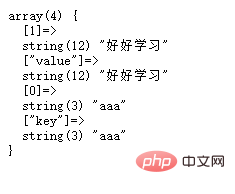```<?php
\$a=[
'aaa'=>'好好学习',
'bbb'=>'天天向上',
'ccc'=>'福如东海'
];
//第一次each
\$data = each(\$a);
echo '<pre>';
var_dump(\$data);
echo '</pre>';
//第二次each
\$data = each(\$a);
echo '<pre>';
var_dump(\$data);
echo '</pre>';
//第三次each
\$data = each(\$a);
echo '<pre>';
var_dump(\$data);
echo '</pre>';
//第四次each
\$data = each(\$a);
echo '<pre>';
var_dump(\$data);
echo '</pre>';
?>```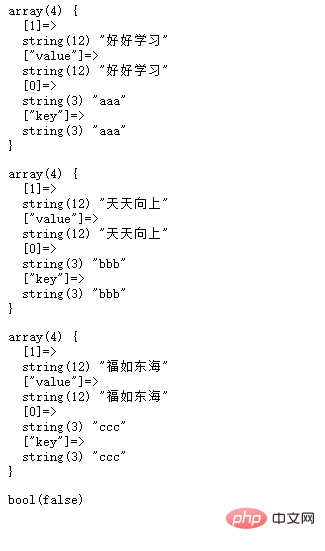list和each的配合

```<?php
\$a=[
'aaa'=>'好好学习',
'bbb'=>'天天向上',
'ccc'=>'福如东海',
];
list(\$key,\$value) = each(\$a);
echo \$key. '-----' .\$value .'<br />';
?>```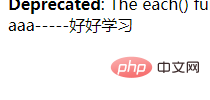```<?php
\$a=[
'aaa'=>'好好学习',
'bbb'=>'天天向上',
'ccc'=>'福如东海',
];
while(list(\$key,\$value) = each(\$a)){
echo \$key. '-----' .\$value .'<br />';

}
?>```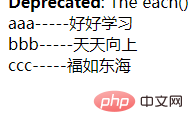• 相关标签：PHP 函数
• https://www.php.cn/php-weizijiaocheng-483105.html